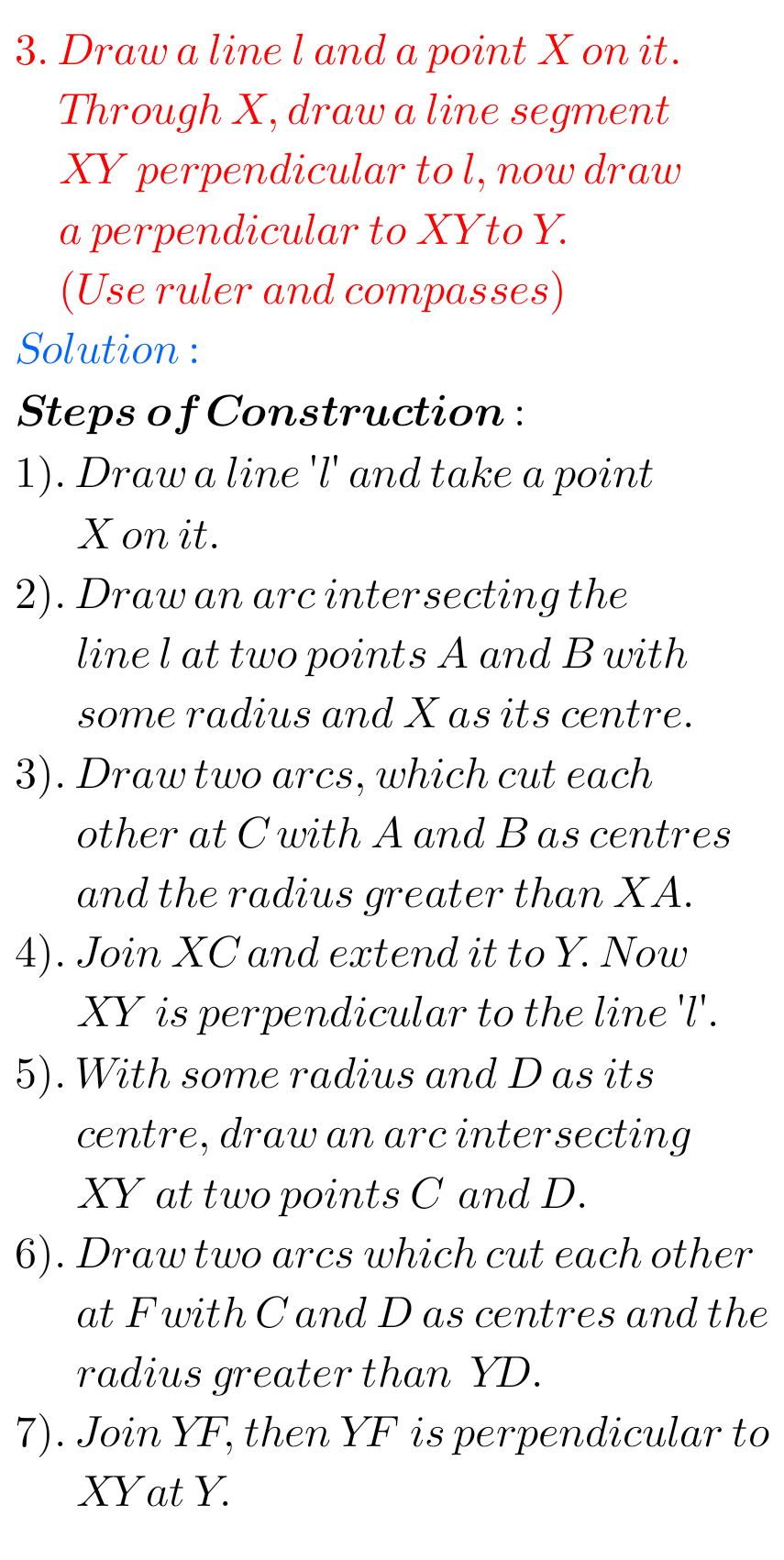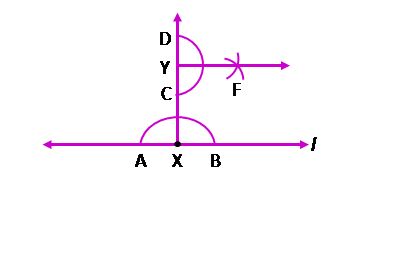## Practical Geometry Solutions Exercise 14.4 Class 6 Ncert

Ncert solutions for Mathematics Class 6th textbook Chapter 14 Practical Geometry Exercise 14.4 are given.

First you study the textbook lesson Practical Geometry very well.

You should also observe the example problem and solutions given in the textbook.

Observe the given below solutions and try them in your own method.

You can see solutions for class 6 Maths Ncert

Chapter 1 Knowing our Numbers

Chapter 2 whole Numbers

Some chapters solutions links are given below.

M

### Maths Solutions for Practical Geometry Exercise 14.4 Class 6 Ncert

Ncert Maths Chapter 14 Practical Geometry Exercise 14.4 solutions

Exercise 14.4### Maths class 6th Solutions Practical Geometry exercise 14.4 NcertNote: Observe the solutions and try them in your iwn method.

Chapter 3 Playing with Numbers

Chapter 4 Basic Geometrical Ideas

Exercise 4.1

Exercise 4.2

Exercise 4.3

Exercise 4.4

Exercise 4.5

Exercise 4.6

Integers

Chapter 6 Integers

Fractions

Chapter 14 Practical Geometry

Exercise 14.1

Exercise 14.2

Exercise 14.3

Exercise 14.4

Exercise 14.5

Exercise 14.6